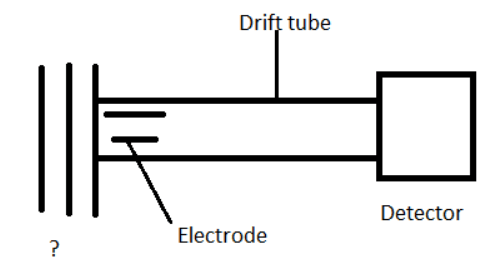# Tandem Mass Spectroscopy

Tandem Mass Spectroscopy

1. Tandem mass spectroscopy combines which of the following devices?

a) Mass spectrometer and gas-solid chromatograph
b) Mass spectrometer and gas-liquid chromatograph
c) Mass spectrometer and gas chromatograph
d) Mass spectrometer and mass spectrometer

Explanation: Tandem mass spectroscopy combines two mass spectrometers. It is represented as MS/MS.

2. In tandem spectroscopy, the first stage sepatation device is a mass spectrometer.

a) True
b) False

Explanation: In tandem spectroscopy, the first stage sepatation device is a mass spectrometer. It is represented as MS/MS.

3. Which of the following is used to separate a single mass that is characteristic of a given analyte in a mixture?

a) First mass spectrometer
b) Second mass spectrometer
c) Filter
d) Precursor

Explanation: First mass spectrometer is used to separate a single mass that is characteristic of a given analyte in a mixture. The single mass is known as the precursor.

4. The mass-selected ions are activated in which of the following ways that causes them to fall apart to produce product ions?

a) Collisional activation
b) Evaporational activation
c) Inert gas activation
d) Thermal activation

Explanation: The mass-selected ions are activated by some means that cause them to fall apart to produce product ions. They are activated by collisional activation.

5. The final MS/MS spectrum consists only of product ions from the selected precursor.

a) True
b) False

Explanation: The final MS/MS spectrum consists only of product ions from the selected precursor. The chemical background and other mixture components are absent.

6. In reverse-geometry mass spectrometer which of the following precedes the electric sector?

a) Nebulizer
b) Orifice
c) Magnetic sector
d) Mass spectrometer

Explanation: In reverse-geometry mass spectrometer magnetic sector precedes the electric sector. Early work on MS/MS was done with reverse-geometry double focussing mass spectrometer.

7. A magnetic sector alone can be used as a mass spectrometer, with roughly _____ resolution.

a) Low
b) High
c) Unit
d) Infinite

Explanation: A magnetic sector alone can be used as a mass spectrometer, with roughly unit resolution. Early work on MS/MS was done with reverse-geometry double focussing mass spectrometer.

8. What does the acronym ‘MIKES’ stand for?

a) Mass-analysed ion kinetic energy spectrometer
b) Mass-based induced kinetic energy spectrometer
c) Mass invasive kinetic electric spectrometer
d) Mass-analyser in kinetic energy-type spectrometer

Explanation: ‘MIKES’ stands for mass-analysed ion kinetic energy spectrometer. It is related to spectrometers having magnetic field sectors.

9. In MIKES experiments which of the following are measured?

a) Product ions
b) Product ion kinetic energies
c) Product ions mass to charge ratio
d) Product ions masses

Explanation: In MIKES experiments, mass to charge ratios are not measured. Product ion kinetic energies are measured in these experiments.

10. All ions with the same number of charges will have __________

a) Same kinetic energy
b) Different kinetic energies
c) Same mass
d) Different mass

Explanation: All ions with the same number of charges will have same kinetic energy. It is assumed that all ions have a single charge.

11. A tandem mass spectrometer has which of the following analysers?

a) Time of flight mass analyser
b) Magnetic deflection analyser

Explanation: A tandem mass spectrometer has a filter. The filter is followed by time of flight mass analyser.

12. Which of the following filters are used in tandem spectrometer?

b) Low energy filter
c) High energy filter
d) Time of flight mass filter

Explanation: A tandem mass spectrometer has a quadrupole mass filter. The filter is followed by an analyser.

13. B/E scan is which of the following scans?

a) Product ion scan
b) Precursor ion scan
c) Mass scan
d) Charge scan

Explanation: B/E ratio can be chosen to select ions with a given velocity. It is a product ion scan.

14. Which of the following is located in the region between two analysers?

a) Nebuliser
b) Collision cell
c) Filter
d) Vacuum chamber

Explanation: Collision cell is located in the region between two analysers. Low energy parent ions are mass analysed.

15. Given below is the diagram of the drift tube of a tandem quadrupole/time of flight instrument. Identify the unmarked component.a) Nebuliser
b) Filter
c) Chopper
d) Acceleration lens